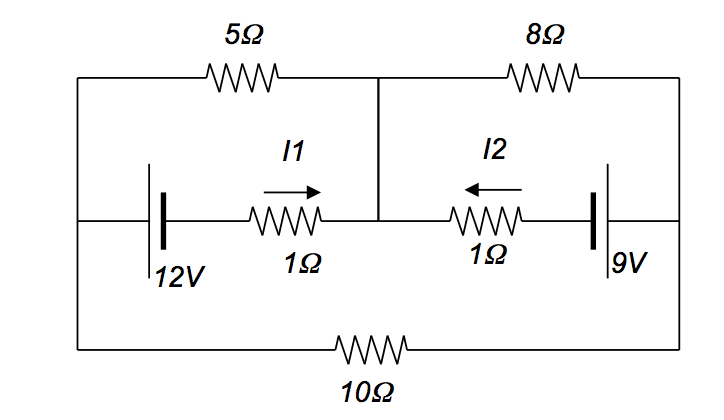# Find currents I1 and I2 in a 3-loop, 2-battery circuit

## Homework Statement

Find currents I1 and I2 in the following circuit:## Homework Equations

I know I have to use Kirchoff's loop rule but I am not sure of the current going through the 5 ohm and 8 ohm resistors, is it just I1 and I2 respectively? Also do I incorporate the large loop at the bottom?

## The Attempt at a Solution

-12 - (1)I1 - (5)I1 = 0
-9 - (1)I2 - (8)I2 = 0
I get negative answers with the above^

This is a practice midterm problem, so it's not my actual homework.[/B]

## Answers and Replies

I haven't checked your arithmetic or if your stated equations are correct, but there is nothing wrong with getting negative values. Since these are linear equations, it only means that the direction of the current is actually going the other way as what was originally assumed.

I know I have to use Kirchoff's loop rule but I am not sure of the current going through the 5 ohm and 8 ohm resistors, is it just I1 and I2 respectively? Also do I incorporate the large loop at the bottom?
As for the answer to that question, think of it this way. If the current going through the 5 ohm resistor were ##I_1##, the bottom loop, with the 10 ohm resistor, would not have any current going through it. Can you see why? Apply Kirchhoff's junction/node rule to junction on the left, and you'll see there have to be three currents... one going through the 10 ohm resistor, your ##I_1##, and whatever current is going through the 5 ohm resistor.

gneill
Mentor
Hi prajakta,

Welcome to Physics Forums!

The currents I1 and I2 refer to just the currents in the components that they are next to. Other components will have different current values. Certainly the currents through the 5 and 8 Ohm resistors will not be equal to I1 and I2.

You can apply Kirchhoff's current law (KCL) throughout the circuit, defining "new" currents as required until every component is accounted for. Then you'll be in a position to use KVL to write loop equations and solve for all the currents.

Last edited:
•scottdave
scottdave
Science Advisor
Homework Helper
Sorry, my previous answer was considered too much help. Just know that you will need 3 loop equations, and all of the components need to be included at least once. Please note that I1 and I2 combine at the node, and the only think you know for sure is that all of this current will go to either the 8 ohm or the 5 ohm. Do not assume that the current through the 5 ohm is the same as i1.
As another poster stated, you can use I1 to represent the current through the 1ohm, but the current through the 5 ohm will be some combination of I1 and another current, from the 3rd loop. Similar with the 8ohm. I hope this helps. And yes, negative current values are OK. It just means that the actual current is flowing in the opposite direction of the assumed direction.

Last edited: